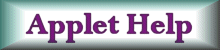Relationship between simple harmonic motion and constant circular motion. Physical meaning of the phase. Topics:  Simple harmonic motion, circular motion, rotational kinematics, Hooke's law, spring force.Pre-requisite skills:  Basic vector algebra. Approximate completion time:  Under an hour. Provide sufficient detail to verify that the assignment was completed in a meaningful manner.Applet by Angel Franco Garcia 1.  Run the applet by pressing the "Start" ("Empiaza") button. Familiarize yourself with the operation of the applet. The applet is broken into three major parts. The first shows a particle undergoing circular motion at a constant rate. The second shows the same particle on a vertical spring. Finally, the third plots the time evolution of the system -- the result is a sine wave. Consider the positions of the two particles at any time during the motion.  (You can press the "Pause" ("Pausa") button to freeze the motion if you find this helpful.) (a) What is the relationship between the displacement vectors of the two particles? (b) What is the relationship between the velocity vectors of the two particles? (c)  Given your answers to parts (a) and (b), can you guess how the acceleration vectors of the two particles are related? (d)   By examining your answers to the previous questions, summarize an important way in which constant circular motion is related to simple harmonic motion. 2. In physics, we often use the term "angular frequency" (or "angular speed") to describe the frequency of oscillation of a particle on a spring, even though the particle is moving along a straight line. (We even use the same notation, w, and the same units, radians per second.) (a) By examining the applet as it is running, describe why we can apply the angular frequency of the particle undergoing constant circular motion to the particle on the spring. 3.  Set the frequency f = 1.  As the applet runs, notice that the applet generates a plot of a sine wave on the far right. (a)  What do the horizontal and vertical axes of the plot represent in terms of the motion of the two particles? (b) Where in the motion of the particle on the spring is the speed a maximum?  (c) Where in the motion of the particle on the spring is the speed a minimum?  (d) What points on the sine wave refer to the minimum and maximum speeds?  How do the slopes compare at these points? 4.  Now we will consider what happens when the values of the following parameters are changed: (a)  The Amplitude (i) What happens to the frequency of oscillation when the amplitude is changed?  (ii) Is this surprising?  Why or why not? (b) The Frequency (i) What happens to the amplitude of oscillation when the frequency is changed? (ii)  Notice that the wavelength changes when the frequency changes.   If the frequency is doubled, does the wavelength get larger or smaller? By exactly how much? (c) The Initial Phase ("Fase Inicial") (i) Describe how changing the phase affects the following (if at all): the amplitude, the wavelength, the frequency. (ii) What is the physical significance of the phase in terms of the initial position of the particle on the spring? (iii) Which value of the phase turns the sine wave into a cosine wave?  What does this mean physically? 5.  Now consider the particle on the spring. (a) When it is in its equilibrium position (that is, at x = 0), what is the net force acting on the particle?   Therefore, what would be the acceleration at this point?  (b) By considering the net force acting on the particle at various positions, state where in the motion of the particle on the spring the acceleration is a maximum. Helpful Resources Circular Motion by Zona Land. Oscillation and Wave by Fu Kwun Hwang. Simple Harmonic Motion and Uniform Circular Motion by Fu Kwun Hwang. Circular Motion and Simple Harmonic Motion by Sadahisa Kamikawa. Circle and Something More by Surendranath Reddy B. Amplitude of Circular Motion by Angel Franco Garcia. Return to Web Assignments